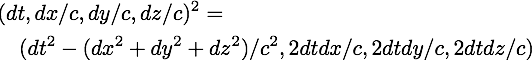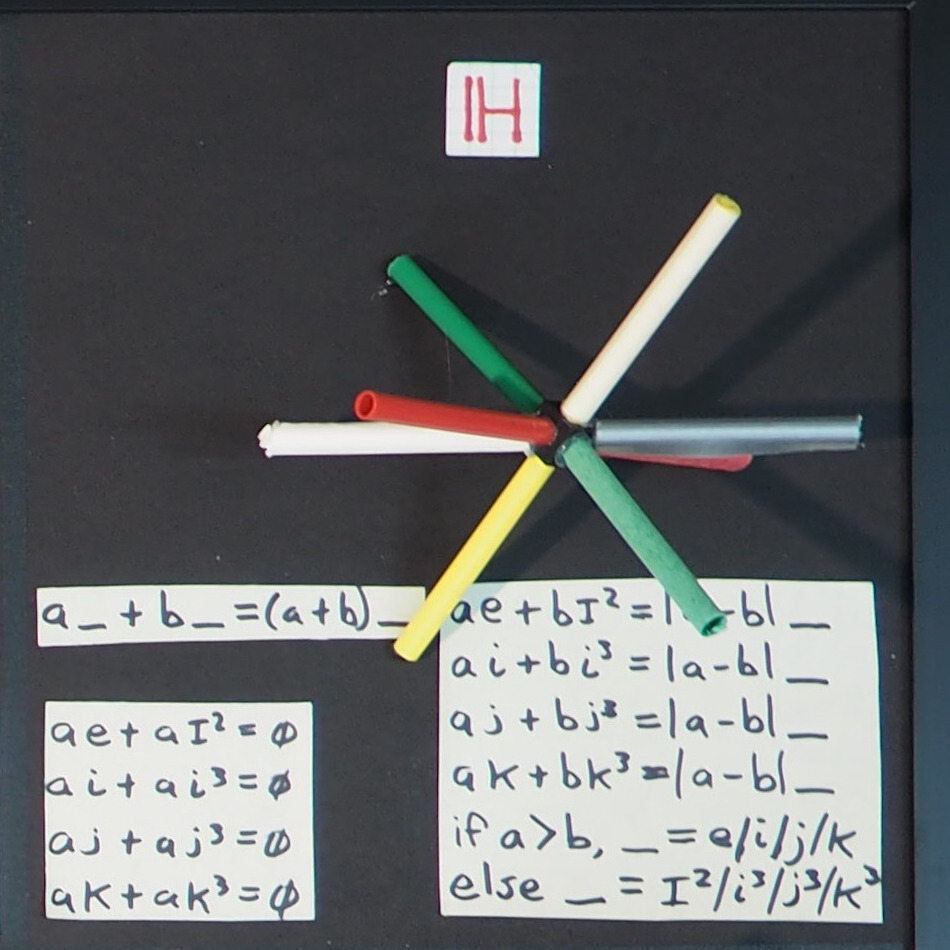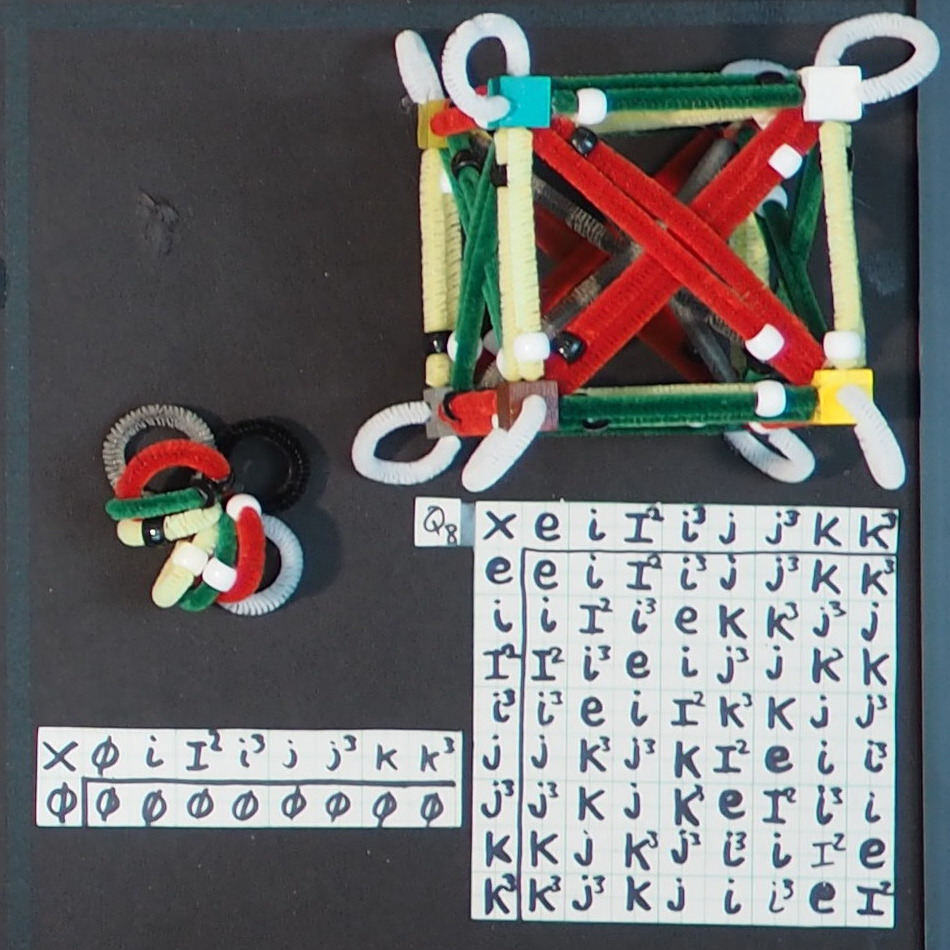I gave a 15 minute talk at a local Americal Physical Society Meeting.  Here is the title and abstract.

Title: A Unified Mathematical Field Theory

Abtract: The century old problem of getting gravity to play gracefully with the rest of physics may not have to do with a physics field theory. Instead it may have to do with the math tools used for any physics field theory. By third grade, we had all learned how to add, subtract, multiply, and divide any number. By the end of physics graduate school, the tools of differential geometry no longer allow one such freedom to play with expressions. Here is how one calculates the interval between two events in flat space-time using a metric tensor and a 4-vector:This looks like a square. Design an algebra (similar to quaternions) that removes the Greek letters along with the metric tensor:The first term is known as the Lorentz invariant interval of special relativity. Two inertial observers will agree to that. This expression has more information than just the interval. The other three terms I call space-times-time. Having two observers agree about space-times-time but disagree about the interval may be the new math for gravity.

Note: the title is meant to be a variation on "Unified Field Theory".  The numbers discussed do not commute and thus are not a mathematical field that must commute by definition. A more precise title would have been "Unified Division Algebra Theory", but that does not carry the same punch.

If this abstract is of interest to you, see:

The 15 slides - http://bit.ly/vp-umft

A 22 minute presentation of those slides - http://bit.ly/vp-umftv

There were about a dozen people in the room. It was the last contributed talk of the meeting. The only question I got concerned how I did an animation.

No one solves hard problems all by themselves. And certainly no one whose chosen label is "the low fidelity ultra-orthodox fringe" will be able to pull this off. One issue I don't get is the tension between treating measurements in space-time as one cohesive, indivisible number and the world of 4-dimensional vector spaces over a mathematical field such as the real numbers. There are nine sets, none of which is a mathematical field. The unitary group for zero connects them creating a star topology for addition:This kind of topology isn't part of the discussion with a four-dimensional vector space where all are independent of each other.

The rules for multiplication come right out of group theory instead of Gauss/Hamilton/Rodrigues making them up. Here is the graph theory for it:Zero looks like a spider. Non-zero multiplication looks insane. When I get pressed about describing space-time numbers as a 4-vector, it just sounds like they are skipping over the richness that is here.

The answer I seek might be in the mathematics literature somewhere. It would involve complex numbers on a complex manifold and the Abelian cyclic group Z4 for the rules of multiplication. It would not use the real numbers. A discussion of the relationship between Z4 and coordinate transformations is what is of interest to me. That would be a start, since one would swap the groups from Z4 to Q8. But that is only a start. Then you face a far deeper issue: how do you do differential calculus with the provided rules for addition and multiplication? I have read a few technical papers on the subject (Sudbery_1, Sudbery_2, Deavours). Since none of these efforts can show that a function like q2 is analytic in q, I consider them to be failures. A wizard in number theory who knows quantum field theory may be required. That is a rare creature indeed.